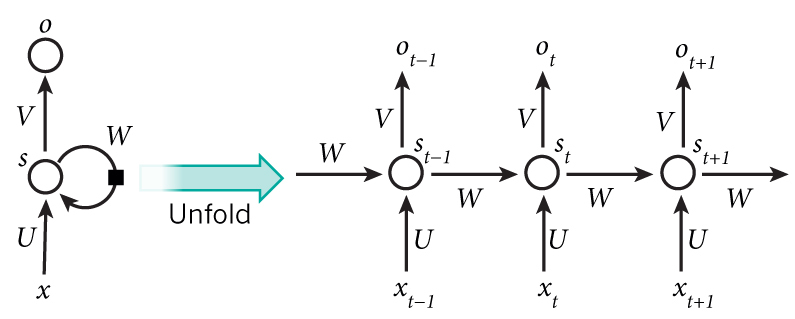March 18th, 2019

### Days 71 - 73: Recurrent Neural NetworksBy Aidin

Here, I summarize some of the famous recurrent neural network (RNN) architectures.

An RNN processes sequences, for instance, daily stock prices, sentences, or sensor measurements — one element at a time while retaining a memory (called a state) of what has come previously in the sequence. The general architecture for an RNN is as follows:An RNN finds the temporal dependencies in the input data thus can be used for forecasting.

RNNs are different from recursive neural networks or we can say that recursive neural networks are the general or parent models of RNNs. In a recursive network, the weights are shared (and dimensionality remains constant) at every node for the same reason. This means that all the W_xh weights will be equal(shared) and so will be the W_hh weight. This is simply because it is a single neuron which has been unfolded in time. A recursive neural network generally looks as follows:It is quite simple to see why it is called a Recursive Neural Network. Each parent node's children are simply a node similar to that node.

Another famous RNN architecture is called long short-term memory (LSRM) cell. The architecture looks as follows generally:• LSTM is a novel recurrent network architecture training with an appropriate gradient-based learning algorithm.
• LSTM is designed to overcome error back-flow problems. It can learn to bridge time intervals in excess of 1000 steps.
• This true in the presence of noisy, incompressible input sequences, without loss of short time lag capabilities.

A common LSTM unit is composed of a cell, an input gate, an output gate and a forget gate. The cell remembers values over arbitrary time intervals and the three gates regulate the flow of information into and out of the cell.

Another famous architecture is called gated recurrent units (GRU). The GRU is like a long short-term memory (LSTM) with forget gate but has fewer parameters than LSTM, as it lacks an output gate. GRU's performance on certain tasks of polyphonic music modeling and speech signal modeling was found to be similar to that of LSTM. GRUs have been shown to exhibit even better performance on certain smaller datasets. The following is the general architecture of a GRU:But note that the GRUs are shown to be generally weaker than LSTMs.

In addition, a Neural Turing machine (NTMs) is a recurrent neural network model published by Alex Graves et. al. in 2014. NTMs combine the fuzzy pattern matching capabilities of neural networks with the algorithmic power of programmable computers. An NTM has a neural network controller coupled to external memory resources, which it interacts with through attentional mechanisms. The memory interactions are differentiable end-to-end, making it possible to optimize them using gradient descent.